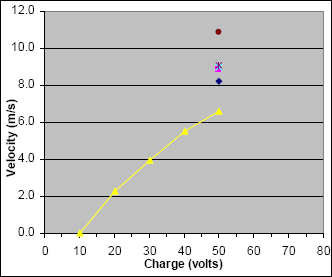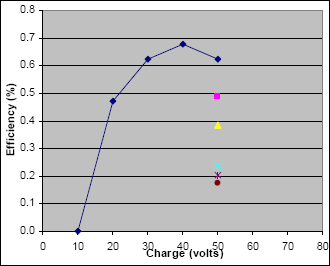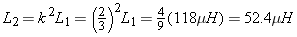# Coil of 84 Turns

This was the second coil tested, smaller than the first coil tested by 13 turns.

## Coil of 84 Turns

See the Projectiles page for details of these projectiles.

### Measurements

The raw measurements are shown in red in the table below, using horizontal ballistics measurements. The symbol "-" indicates that it was not attempted, and "dnf" means "did not fire".

Projectile:

A
C
D
E
F
G
Length:   3.5" 2.5" 2" 1.75" 1.5" 1.25"
Mass:   4.301 g 2.163 g 1.464 g 0.8722 g 0.7345 g 0.4421 g
Potential
energy
(joules)

Charge
(volts)
Horiz
Distance
(inches)
Horiz
Distance
(inches)
Horiz
Distance
(inches)
Horiz
Distance
(inches)
Horiz
Distance
(inches)
Horiz
Distance
(inches)
0.6 J 10 v dnf - - - - -
2.4 20 40 - - - - -
5.4 30 69 - - - - -
9.6 40 96 - - - - -
15.0 50 115 144 155 157 159 190

### Velocity

Again using the equations for horizontal ballistics and the raw data, the velocity is calculated for each of the measurements above and is shown in red.

Projectile:

A
C
D
E
F
G
Potential
energy
(joules)

Charge
(volts)

Velocity
(m/s)

Velocity
(m/s)

Velocity
(m/s)

Velocity
(m/s)

Velocity
(m/s)

Velocity
(m/s)
0.6 J 10 v 0.0 - - - - -
2.4 20 2.289 - - - - -

5.4
30 3.949 - - - - -
9.6 40 5.494 - - - - -
15.0 50 6.581 8.241 8.871 8.985 9.100 10.874

### Efficiency

Calculate efficiency as shown in red.

Projectile:

A
C
D
E
F
G
Potential
energy
(joules)

Charge
(volts)

Efficiency
(%)

Efficiency
(%)

Efficiency
(%)

Efficiency
(%)

Efficiency
(%)

Efficiency
(%)
0.6 J 10 v 0.0 % - - - - -
2.4 20 0.5 - - - - -
5.4 30 0.6 - - - - -
9.6 40 0.7 - - - - -
15.0 50 0.6 0.5 0.4 0.2 0.2 0.2

### Graphical Results### Coil Analysis

This coil with 84 turns measured 6 ms half-cycle waveform (an oscilloscope image is not available).

The 6 ms timing is a good result compared to predictions from using the approximate air-core inductor model. This confirms our approach (applying a percentage change from air-core inductance to an iron-core model) is useful.

### How Does This Coil Need to Change?

The speeds and efficiencies are still very low. We wish to reduce the time from 6 ms by 33%, to reach 4 ms discharge time.

We will again apply a percentage change from an air-core approximation to our iron-core physical coil.

1. Using the Java Inductor Simulator again with values of 12 AWG, ID=6mm, OD=60mm, len=16mm, we find that our inductance would be L = 118 uH for an air-core coil.
2. We want a 1/3 smaller LC time constant (i.e. 6 ms becomes 4 ms)3. The new coil in air should therefore have L = 52.4 uH
4. The Java Inductor Sim suggests a 70 turn coil will have the desired inductance.
5. Therefore, removing 14 turns should result in a 4 ms discharge time.

### Damping Resistor

Note the damping resistor will likely need to be changed. As the LC time constant becomes smaller, the resistor also should be reduced to remain at the critically-damped point. Without any resistor change, the tuned circuit will be over-damped and the waveform is lower and longer than desired.

We also found an unexpected and fascinating behavior of this resistor's construction, which required a slight redesign as described in the next page about damping resistor construction.

 < Previous Page 31 of 37 Next >